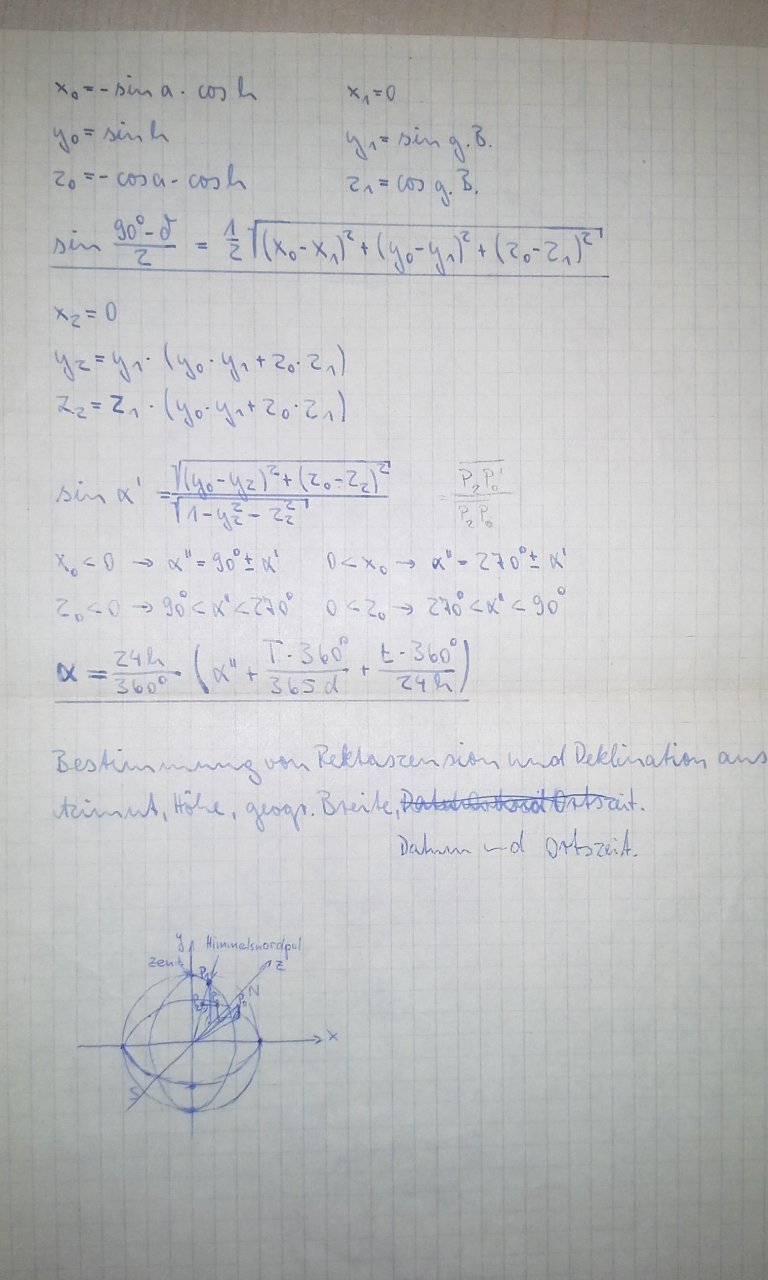Calculation of right ascension and declination from
azimuth, elevation, latitude, date and time

This is something what I did when I just turned 16 years old,  ☞
it took me a few days to figure it out but finally I came up with a formula.
And now a few decades later it's time to check if the formula is correct ;)
So here with a script in this web page I implemented the formula and
from some sample data I found on the web it seems the formula works :)
Input:
azimuth (0..360):°
elevation (0..90):°
latitude (-90..90):°
days since vernal equinox
~March 21 (0..365.25):
d
local time: (0..24):
(sun in south at 12:00)
h
Output:
right ascension:°
declination:°Test - comparison with data I found on the web:
An observer O at geogr. latitude beta=50° N and longitude 10° E, on 1991/05/19 at 13:00 UT,
will see a star of right ascension RA=55.8° and declination delta=19.7°
at azimuth az=43.6° and altitude h=53.4°
Source: http://www.geoastro.de/elevaz/basics/index.htm